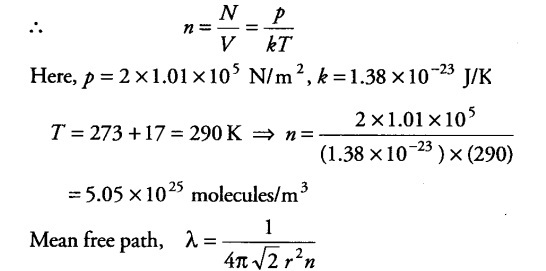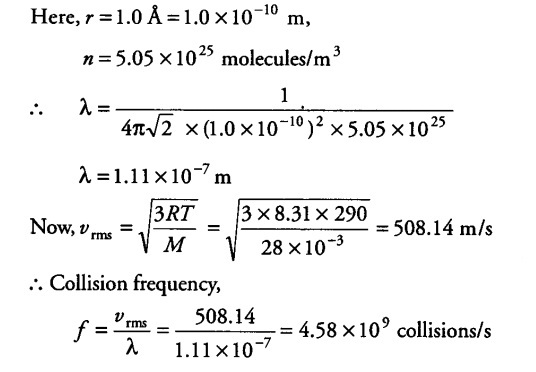# Estimate the mean free path and collision frequency

Estimate the mean free path and collision frequency of a nitrogen molecule in a cylinder containing nitrogen at 2.0 atm and temperature 17°C. Take the radius of a nitrogen molecule to be roughly 1.0 A. Compare the collision time with the time the molecule moves freely between two successive collisions (molecular mass of N2 = 28.0 u)

Let n be the number of molecules per unit volume of the gas.Now, pV = N kT, where N = number of molecules in volume V of the gas